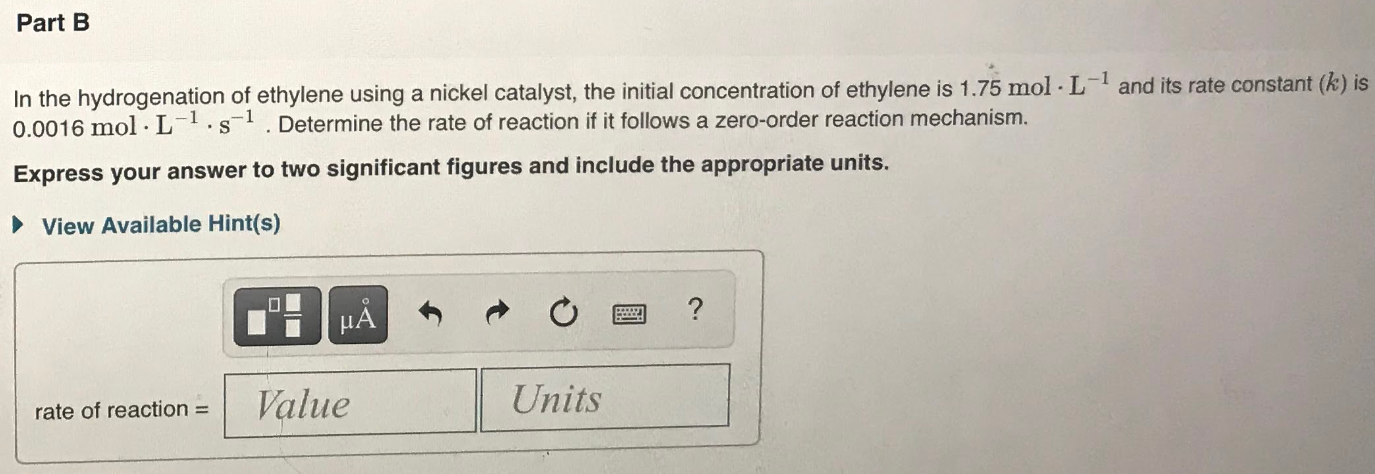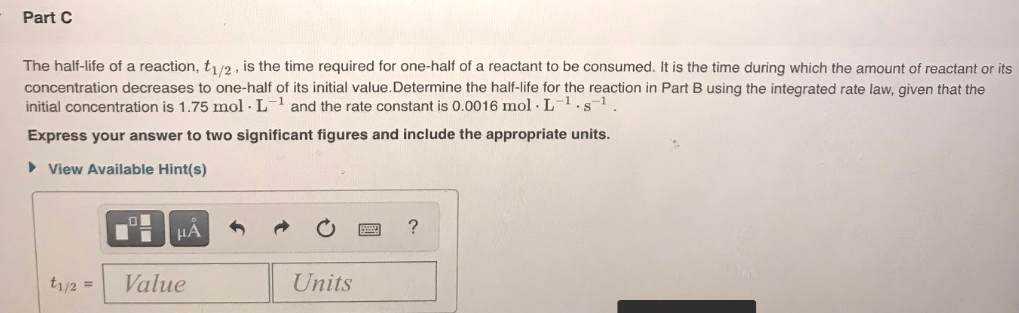# In the hydrogenation of ethylene using a nickel catalyst, the initial concentration of ethylene is 1.75 mol • L^-1 and its rate constant (k) is 0.0016 mol • L^-1 • s^-1. Determine the rate of reaction if it follows a zero-order reaction mechanism. Express your answer to two significant figures and include the appropriate units. The half-life of a reaction, t 1/2, is the time required for one-half of a reactant to be consumed. It is the time during which the amount of reactant or its concentration decreases to one-half of its initial value. Determine the half-life for the reaction in Part B using the integrated rate law, given that the initial concentration is 1.75 mol • L^-1 and the rate constant is 0.0016 mol • L^-1 • s^-1. Express your answer to two significant figures and include the appropriate units.